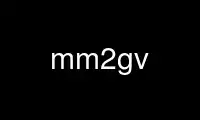# mm2gv - Online in the CloudThis is the command mm2gv that can be run in the OnWorks free hosting provider using one of our multiple free online workstations such as Ubuntu Online, Fedora Online, Windows online emulator or MAC OS online emulator

### PROGRAM:

#### NAME

mm2gv - Matrix Market-DOT converters

#### SYNOPSIS

mm2gv [ -cluv? ] [ -Ui ] [ -ooutfile ] [ file ]

#### DESCRIPTION

mm2gv converts a sparse matrix of the Matrix Market format to a graph in the GV (formerly
DOT) format. If the matrix M is not square, the graph is considered bipartite and the
matrix is viewed as a bipartite graph adjacency matrix, with the rows and columns of the
matrix specifying the two sets of vertices. Equivalently, the matrix is converted into a
symmetric square matrix

0 M
\$M sup T\$ 0
a block matrix with square blocks of 0's in the upper left and lower right, the upper
right block being M and the lower left block being the transpose of M. This matrix is
then viewed as the adjacency matrix of the graph.

For a square matrix, mm2gv uses it directly as an adjacency matrix if its pattern of non-
zero entries is symmetric; otherwise, it will treat it as a bipartite graph as with the
case of non-square matrices. This behavior can be modified by the -U flag.

#### OPTIONS

The following options are supported:

-c This flag causes mm2gv to assign colors to the edges. The matrix element is scaled
to the range [0,1] depending on where it lies between the minimum and maximum set
matrix values. This scaled value is used as the "wt" attribute of the corresponding
edge. In addition, this scalar value is mapped to an RGB value, which is stored as
the edge "color".

-l If set, mm2gv attaches a label to the graph indicating the base name of the input
file, and the number of nodes and edges.

-u If specified, the graph is assumed to be undirected. By default, the graph
generated is directed.

-v This flag causes mm2gv to store the matrix values as the "len" attribute of the
corresponding edge.

-Ubflag
Specifies how square matrices are handled. If bflag is 0, a square matrix will
always be treated as an adjacency matrix. If bflag is 1 (the default), a square
matrix with a symmetric pattern of non-zero entries will be used as an adjacency
matrix; otherwise, it will be used a bipartite graph. If bflag is 2, a symmetric
matrix will be used as an adjacency matrix; otherwise, it will be used a bipartite
graph. If bflag is 3, any input matrix will be treated like a bipartite graph.

-ooutfile
Prints output to the file outfile. If not given, mm2gv uses stdout.

OPERANDS
The following operand is supported:

file Name of the file in MatrixMarket format. If no file operand is specified, the
standard input will be used.

#### RETURNCODES

Return 0 if there were no problems during conversion; and non-zero if any error occurred.

#### AUTHORS

Yifan Hu <yifanhu@research.att.com>
Emden R. Gansner <erg@research.att.com>

See http://math.nist.gov/MatrixMarket/ for description of the format and
http://www.cise.ufl.edu/research/sparse/matrices/ for a large collection of sparse
matrices in this format.

31 July 2008 MM2GV(1)

Use mm2gv online using onworks.net services

Free Servers & Workstations